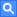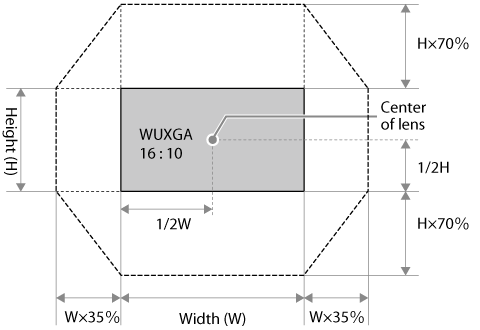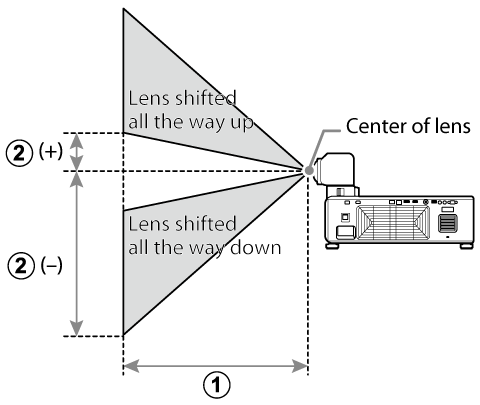Enter a search term and click.

# Projection Distance

## Lens Shift Range

### Lens Shift Range: Landscape Orientation## Projection Distance

### Landscape Orientation

More details on projection distance are available via a simulator on the Fujifilm website:

https://optics.fujifilm.com/projector/simulator/en.htmlA Projection distance (cm/in), minimum (projector zoomed all the way out) to maximum (projector zoomed all the way in)

B Vertical distance from center of lens to bottom of screen (cm/in)

### Projection Distance Tables

Screen dimensions (16∶10) A Projection distance (cm/in.) min.–max. zoom B Vertical shift (cm/in.) lowest to highest
Diagonal (in.) W × H (cm/in.)
70 151 ×  94 /
59.4 ×  37.0
50 –  55 /
19.7 – 21.7
−113 to +19 /
−44.5 to  +7.5
80 172 × 108 /
67.7 ×  42.5
58 –  64 /
22.8 – 25.2
−129 to +22 /
−50.8 to  +8.7
90 194 × 121 /
76.4 ×  47.6
65 –  72 /
25.6 – 28.3
−145 to +24 /
−57.1 to  +9.4
100 215 × 135 /
84.6 ×  53.1
72 –  80 /
28.3 – 31.5
−162 to +27 /
−63.8 to +10.6
120 258 × 162 /
101.6 ×  63.8
87 –  96 /
34.3 – 37.8
−194 to +32 /
−76.4 to +12.6
150 323 × 202 /
127.2 ×  79.5
109 – 121 /
42.9 – 47.6
−242 to +40 /
−95.3 to +15.7
200 431 × 269 /
169.7 × 105.9
147 – 161 /
57.9 – 63.4
−323 to +54 /
−127.2 to +21.3
250 538 × 337 /
211.8 × 132.7
184 – 202 /
72.4 – 79.5
−404 to +67 /
−159.1 to +26.4
300 646 × 404 /
254.3 × 159.1
221 – 243 /
87.0 – 95.7
−485 to +81 /
−190.9 to +31.9
Screen dimensions (16∶9) A Projection distance (cm/in.) min.–max. zoom B Vertical shift (cm/in.) lowest to highest
Diagonal (in.) W × H (cm/in.)
70 155 ×  87 /
61.0 ×  34.3
52 –  57 /
20.5 – 22.4
−111 to  +24 /
−43.7 to  +9.4
80 177 × 100 /
69.7 ×  39.4
59 –  65 /
23.2 – 25.6
−127 to  +28 /
−50.0 to +11.0
90 199 × 112 /
78.3 ×  44.1
67 –  74 /
26.4 – 29.1
−143 to  +31 /
−56.3 to +12.2
100 221 × 125 /
87.0 ×  49.2
75 –  82 /
29.5 – 32.3
−159 to  +35 /
−62.6 to +13.8
120 266 × 149 /
104.7 ×  58.7
90 –  99 /
35.4 – 39.0
−191 to  +42 /
−75.2 to +16.5
150 332 × 187 /
130.7 ×  73.6
113 – 124 /
44.5 – 48.8
−239 to  +52 /
−94.1 to +20.5
200 443 × 249 /
174.4 ×  98.0
151 – 166 /
59.4 – 65.4
−318 to  +69 /
−125.2 to +27.2
250 553 × 311 /
217.7 × 122.4
189 – 208 /
74.4 – 81.9
−398 to  +86 /
−156.7 to +33.9
300 664 × 374 /
261.4 × 147.2
227 – 250 /
89.4 – 98.4
−477 to +104 /
−187.8 to +40.9
Screen dimensions (4∶3) A Projection distance (cm/in.) min.–max. zoom B Vertical shift (cm/in.) lowest to highest
Diagonal (in.) W × H (cm/in.)
60 122 ×  91 /
48.0 ×  35.8
49 –  54 /
19.3 – 21.3
−110 to +18 /
−43.3 to  +7.1
70 142 × 107 /
55.9 ×  42.1
57 –  63 /
22.4 – 24.8
−128 to +21 /
−50.4 to  +8.3
80 163 × 122 /
64.2 ×  48.0
65 –  72 /
25.6 – 28.3
−146 to +24 /
−57.5 to  +9.4
90 183 × 137 /
72.0 ×  53.9
74 –  81 /
29.1 – 31.9
−165 to +27 /
−65.0 to +10.6
100 203 × 152 /
79.9 ×  59.8
82 –  91 /
32.3 – 35.8
−183 to +30 /
−72.0 to +11.8
120 244 × 183 /
96.1 ×  72.0
99 – 109 /
39.0 – 42.9
−219 to +37 /
−86.2 to +14.6
150 305 × 229 /
120.1 ×  90.2
124 – 137 /
48.8 – 53.9
−274 to +46 /
−107.9 to +18.1
200 406 × 305 /
159.8 × 120.1
166 – 183 /
65.4 – 72.0
−366 to +61 /
−144.1 to +24.0
240 488 × 366 /
192.1 × 144.1
200 – 220 /
78.7 – 86.6
−439 to +73 /
−172.8 to +28.7

bTIP

Figures are approximate, differing from the actual values by a few percent.